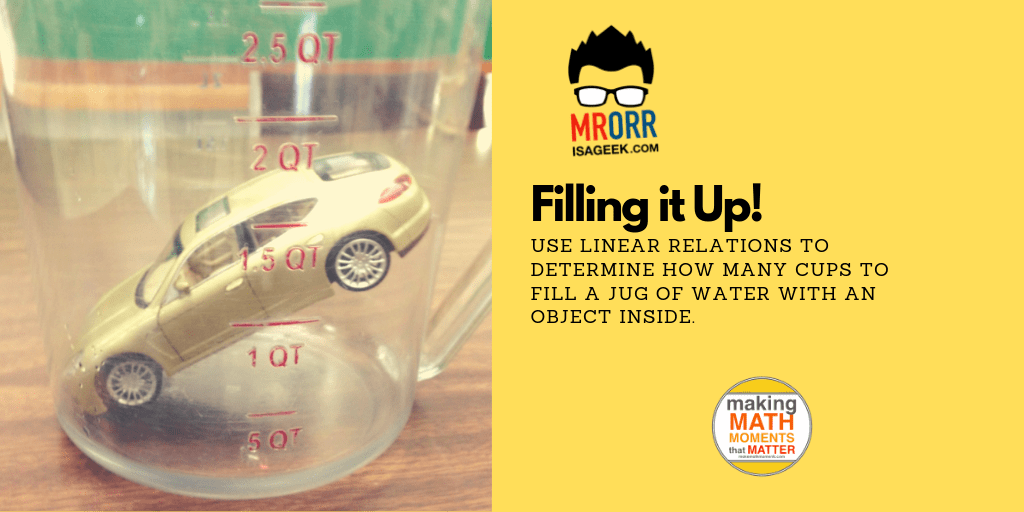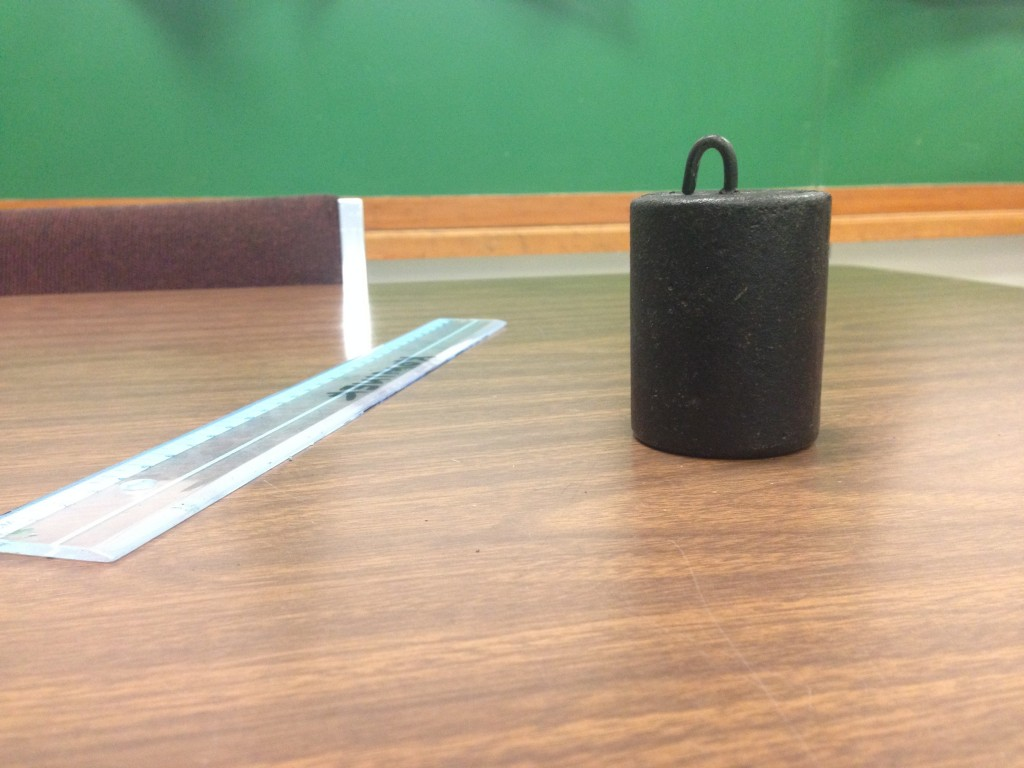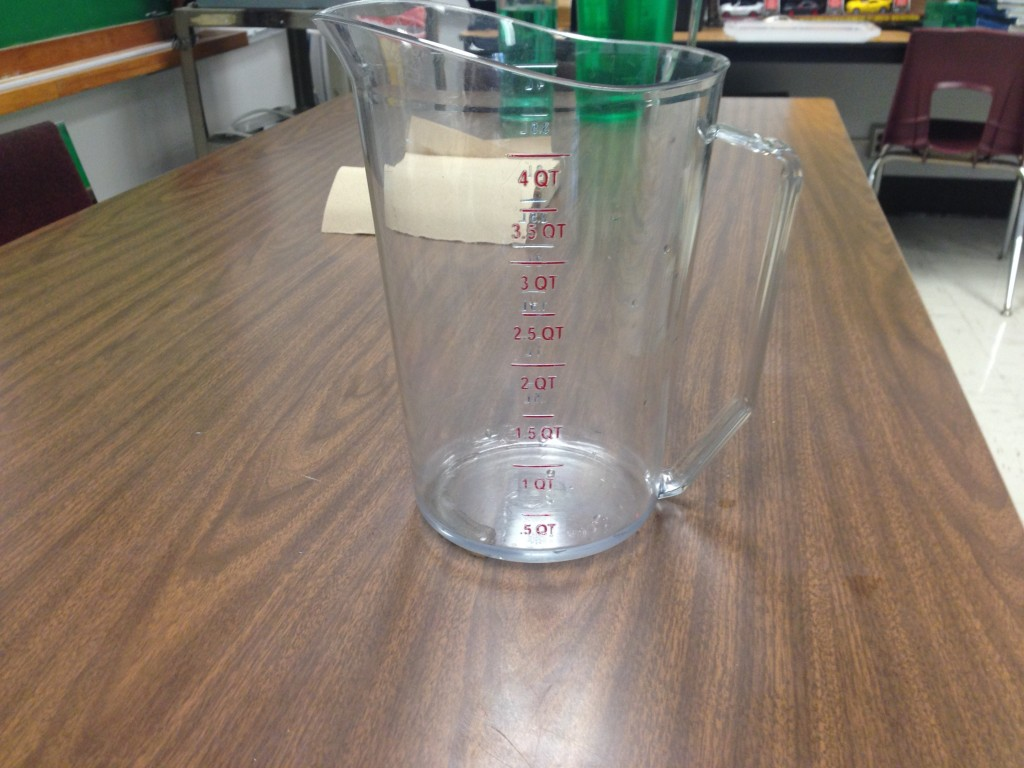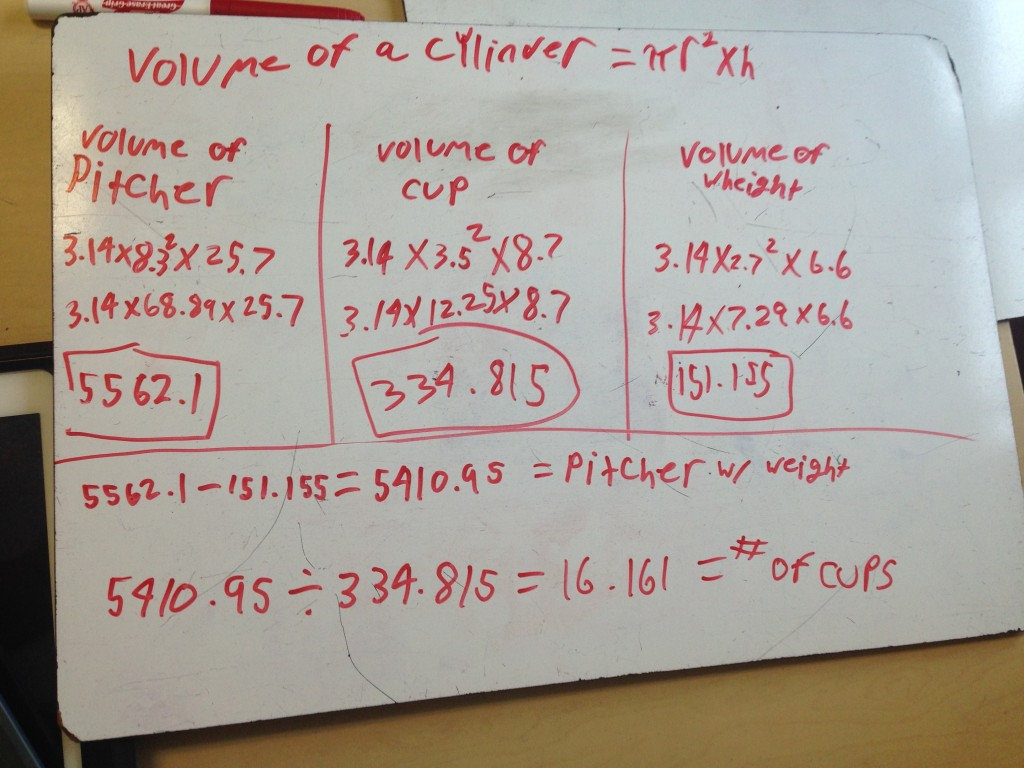# Filling it up!

In our grade 9 applied class we are finishing off linear relations and moving into solving equations. I want an activity that is hands-on, engaging, and shows a purpose to solving linear equations.

Here is some thoughts on an activity I want to try. Let me know what you think. Any feedback would be greatly appreciated.

Here it is: Filling it up!!

Show them this picture….Let them wonder, let them ask what that thing in the pitcher is.

ME:

“How many would be too much?”

“How many would be not enough?”

“How many is just right?”

Have them record the guess. “We’ll compare our answer to our guess”

Next,

ME: Let’s find out how many.

Organize them into groups of 3.

ME: What are we going to need?

We’ll need volume of the pitcher, volume of the cup, and volume of the weight.

Have discussion on:

What shape is the cup? …..is it more like a cylinder or a cone? Which is it closest to? What formula for volume will you use? Will you be right?

What shape is the pitcher? What shape is the weight?

Choose 1 member of you group to find the volume of the cup; choose 1 member for volume of the pitcher; choose 1 member to find volume of the weight.

Have the items around the room like stations:Each member will find the volume of their object and bring it back to the group.

Allow the students to work

Here are some scaffolding questions I can use (Please feel free to give me some more)

• What’s changing as you fill up the pitcher?
• What volume is left after the weight?

Here is a possible solution….My idea is this could be great context for introducing solving equations using opposite operations! Use their technique  and show how the volume grows as the cups increase. Use Desmos and relate it to y = mx + b.show them how their strategy is the same as solving 5562 = 1511 + 335x. Boom! Context for solving equations!

My ideas for extensions would be to put objects like….in the pitcher. Count how many cups to fill the pitcher now. Use our equation to solve for the volume of the car. [Corresponding Grade 9 Academic learning goal: Find the y-intercept (initial value) of a linear equation given the slope (rate of change) and a point. ]

What do you think?? Think it would work? I would love some feedback!